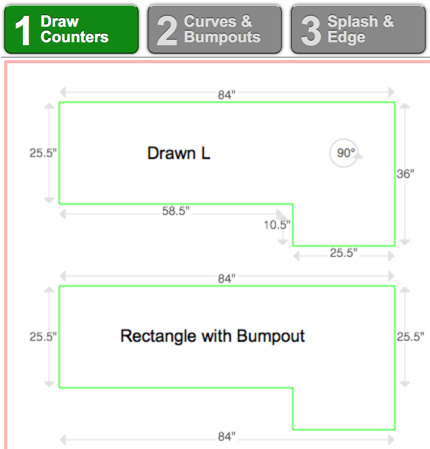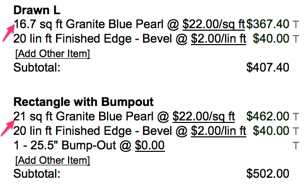# How is Square Footage (Area & Waste) Calculated

### Details on Area Calculation

• For curves & bump-outs, a rectangular box is created and the entire box's sq. ft. is included.
• For inside diagonals, we create a square in the corner to calculate the sq. ft.
• For 22.5, 45, and 67.5 degree angles, we create boxes thereby double-counting area in the angles.
• For L-shapes, we use the actual sq. ft - we do not double count the overlap.

### Tips & Hints

How a countertop is drawn can impact square footage calculation. Most of the time, the simplest way to draw a counter is best. However, sometimes, it might be useful to understand how certain shapes are calculated - particularly if you want a quote to have more square footage (see example below).  If you want to be most precise about square feet (so that you don't under-price a job), the safest thing to do is price by the slab and make sure your slab sizes are accurate (and note that you can make a slab size smaller if you're using - or making - a remnant).

Example: L-shaped countertop drawn with the drawing tool vs. a rectangle with a bumpout.

The drawn L calculations utilize two rectangles versus the bumpout which utilizes a single rectangle for area calculation.
Note that the linear edge is equivalent, just the material area differs.Did this answer your question? Thanks for the feedback There was a problem submitting your feedback. Please try again later.

Still need help? Contact Us Contact Us